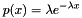#Boost C++ Libraries

...one of the most highly regarded and expertly designed C++ library projects in the world.

## Class template exponential_distribution

boost::random::exponential_distribution

## Synopsis

```// In header: <boost/random/exponential_distribution.hpp>

template<typename RealType = double>
class exponential_distribution {
public:
// types
typedef RealType input_type;
typedef RealType result_type;

// member classes/structs/unions

class param_type {
public:
// types
typedef exponential_distribution distribution_type;

// construct/copy/destruct
param_type(RealType = 1.0);

// public member functions
RealType lambda() const;

// friend functions
template<typename CharT, typename Traits>
friend std::basic_ostream< CharT, Traits > &
operator<<(std::basic_ostream< CharT, Traits > &, const param_type &);
template<typename CharT, typename Traits>
friend std::basic_istream< CharT, Traits > &
operator>>(std::basic_istream< CharT, Traits > &, const param_type &);
friend bool operator==(const param_type &, const param_type &);
friend bool operator!=(const param_type &, const param_type &);
};

// construct/copy/destruct
explicit exponential_distribution(RealType = 1.0);
explicit exponential_distribution(const param_type &);

// public member functions
RealType lambda() const;
RealType min() const;
RealType max() const;
param_type param() const;
void param(const param_type &);
void reset();
template<typename Engine> result_type operator()(Engine &) const;
template<typename Engine>
result_type operator()(Engine &, const param_type &) const;

// friend functions
template<typename CharT, typename Traits>
friend std::basic_ostream< CharT, Traits > &
operator<<(std::basic_ostream< CharT, Traits > &,
const exponential_distribution &);
template<typename CharT, typename Traits>
friend std::basic_istream< CharT, Traits > &
operator>>(std::basic_istream< CharT, Traits > &,
const exponential_distribution &);
friend bool operator==(const exponential_distribution &,
const exponential_distribution &);
friend bool operator!=(const exponential_distribution &,
const exponential_distribution &);
};```

## Description

The exponential distribution is a model of random distribution with a single parameter lambda.

It hasThe implementation uses the "ziggurat" algorithm, as described in

"The Ziggurat Method for Generating Random Variables", George Marsaglia and Wai Wan Tsang, Journal of Statistical Software Volume 5, Number 8 (2000), 1-7.

### `exponential_distribution` public construct/copy/destruct

1. `explicit exponential_distribution(RealType lambda = 1.0);`

Constructs an `exponential_distribution` with a given lambda.

Requires: lambda > 0

2. `explicit exponential_distribution(const param_type & param);`

Constructs an `exponential_distribution` from its parameters

### `exponential_distribution` public member functions

1. `RealType lambda() const;`

Returns the lambda parameter of the distribution.

2. `RealType min() const;`

Returns the smallest value that the distribution can produce.

3. `RealType max() const;`

Returns the largest value that the distribution can produce.

4. `param_type param() const;`

Returns the parameters of the distribution.

5. `void param(const param_type & param);`

Sets the parameters of the distribution.

6. `void reset();`

Effects: Subsequent uses of the distribution do not depend on values produced by any engine prior to invoking reset.

7. `template<typename Engine> result_type operator()(Engine & eng) const;`

Returns a random variate distributed according to the exponential distribution.

8. ```template<typename Engine>
result_type operator()(Engine & eng, const param_type & param) const;```

Returns a random variate distributed according to the exponential distribution with parameters specified by param.

### `exponential_distribution` friend functions

1. ```template<typename CharT, typename Traits>
friend std::basic_ostream< CharT, Traits > &
operator<<(std::basic_ostream< CharT, Traits > & os,
const exponential_distribution & ed);```

Writes the distribution to a std::ostream.

2. ```template<typename CharT, typename Traits>
friend std::basic_istream< CharT, Traits > &
operator>>(std::basic_istream< CharT, Traits > & is,
const exponential_distribution & ed);```

Reads the distribution from a std::istream.

3. ```friend bool operator==(const exponential_distribution & lhs,
const exponential_distribution & rhs);```

Returns true iff the two distributions will produce identical sequences of values given equal generators.

4. ```friend bool operator!=(const exponential_distribution & lhs,
const exponential_distribution & rhs);```

Returns true iff the two distributions will produce different sequences of values given equal generators.